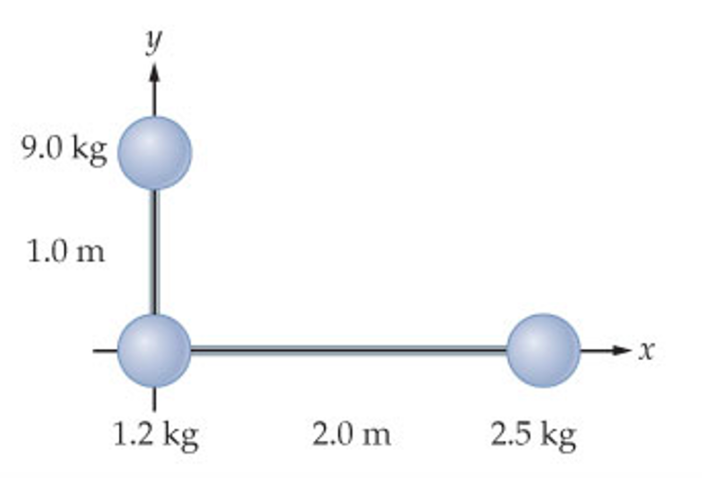# Problem: The L-shaped object in the figure (Figure 1) consists of three masses connected by light rods.What torque must be applied to this object to give it an angular acceleration of 1.00 rad/s2 if it is rotated about the z-axis (which is through the origin and perpendicular to the plane of the figure)?

###### FREE Expert Solution

Moment of inertia of a point mass:

$\overline{){\mathbf{I}}{\mathbf{=}}{\mathbf{m}}{{\mathbf{r}}}^{{\mathbf{2}}}}$

Torque and angular acceleration:

$\overline{){\mathbf{\tau }}{\mathbf{=}}{\mathbf{I}}{\mathbf{·}}{\mathbf{\alpha }}}$

The z-axis passes through the origin and into the page. We have two masses lying outside the z-axis. That is, in the x and y axes.

82% (378 ratings)###### Problem Details

The L-shaped object in the figure (Figure 1) consists of three masses connected by light rods.What torque must be applied to this object to give it an angular acceleration of 1.00 rad/s2 if it is rotated about the z-axis (which is through the origin and perpendicular to the plane of the figure)?